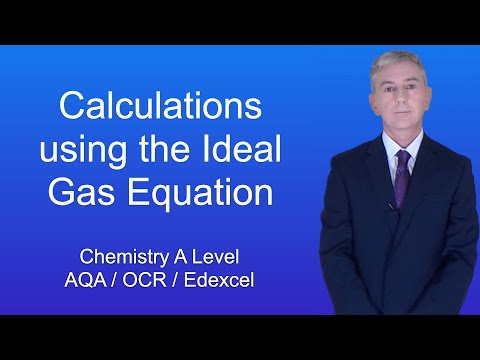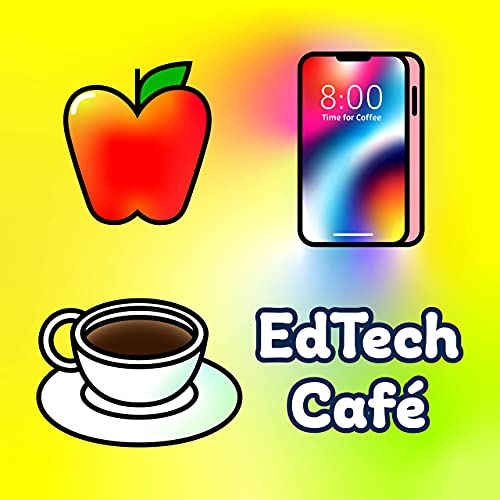# A Level Chemistry Revision “Calculations using the ideal gas equation”

Author: Freesciencelessons via YouTube
Go to Source# EdTech CaféEdTech Café is a podcast series produced by the educational technology team at Stanford Medicine.

In this video, we continue looking at the ideal gas equation. I give you two different questions involving calculations using the idea gas equation. In both questions, you will be required to convert units. This is great practise for using this equation in your exam.

Go to Source

A Level Chemistry Revision “Calculations using the ideal gas equation”
Scroll to top